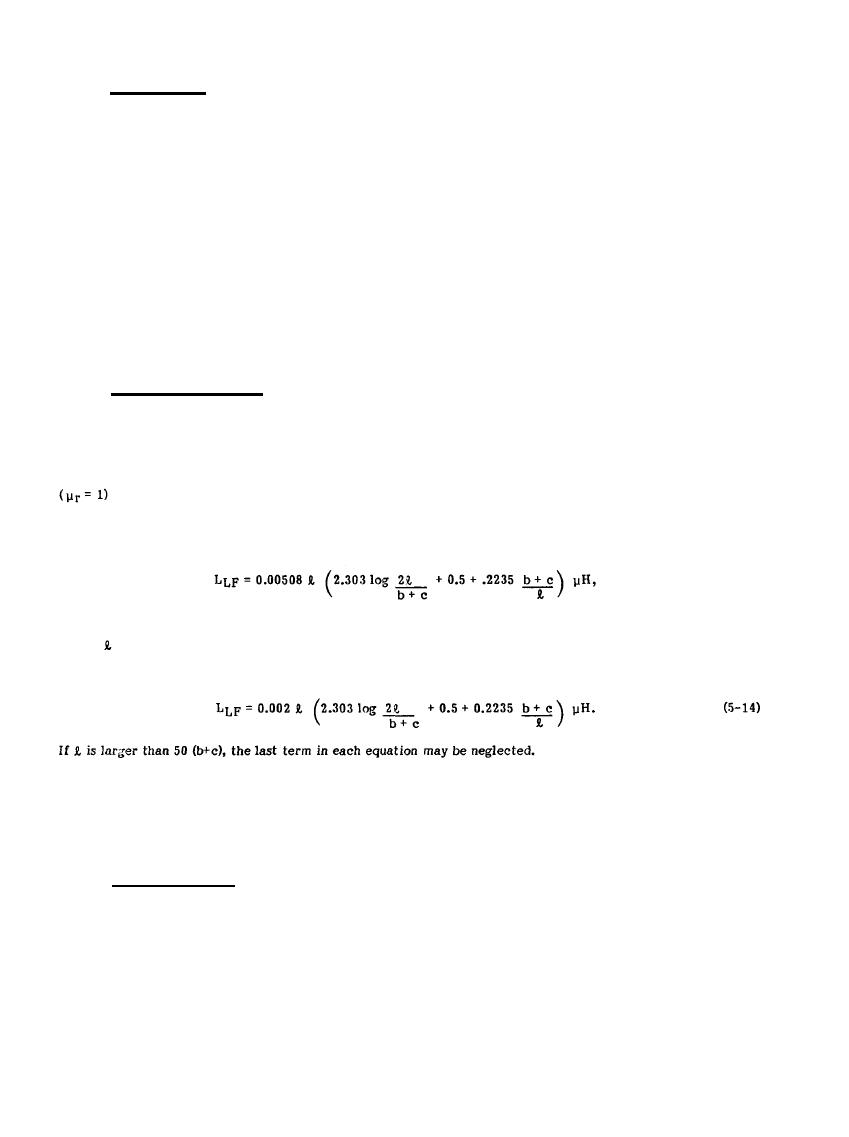Rectangular ConductorsCustom SearchMIL-HDBK-419A
5.2.4.1 Stranded Cable.
A stranded cable consists of a number of wires in close proximity twisted about each other; it is more flexible
than a solid conductor of the same cross-sectional area. Because of the close proximity of the wires, the skin
effect within the cable redistributes most of the current to outer wires. These outer wires are in the form of a
coil (due to the lay of the strand), thus increasing the self-inductance of the cable. Skin effect also increases
the ac resistance as the frequency is increased.
For a given cable size, both the ac resistance and the self-inductance of a stranded conductor are greater than
those of a solid round conductor.  Because of their ineffectiveness at higher frequencies, it has been
recommended that stranded cables not be used at frequencies over 1200 Hz (5-7). However, in many situations,
large cables are required to safely carry currents produced by power faults and lightning discharges; in addition,
solid wires larger than approximately 0.6 cm (0.25 in.) may be difficult to obtain.
5.2.4.2 Rectangular Conductors.
At frequencies high enough to make the skin effect noticeable, the resistance ratio of a flat rectangular
conductor will be lower than that of a solid round wire with the same cross-sectional area if the
width-to-thickness ratio exceeds approximately 2:1. The resistance ratios for several sizes of nonmagnetic
rectangular conductors are plotted in Figure 5-6.
The self-inductance at lower frequencies of a rectangular conductor is (5-6)
(5-13)
where
is the length, b is the width and c is the thickness, and all the dimensions are in inches. For the
dimensions in cm, Equation 5-13 is
The sharp edges on rectangular conductors tend to radiate energy into space and a flat conductor may become
an efficient antenna. To reduce the efficiency of the antenna and minimize this the edges of the
rectangular conductor can be rounded to form an elliptical shape.
5.2.4.3 Tubular Conductors.
Tubular conductors provide the best compromise between factors such as availability, cost weight,
cross-sectional area, skin effect, resistance ratio and inductance. By using the actual cross-sectional area of
the conductive material, the dc resistance of tubular conductors can be determined from Equation 5-1; it is
given for three different diameter copper tubes in Figure 5-7.
5-13Integrated Publishing, Inc.